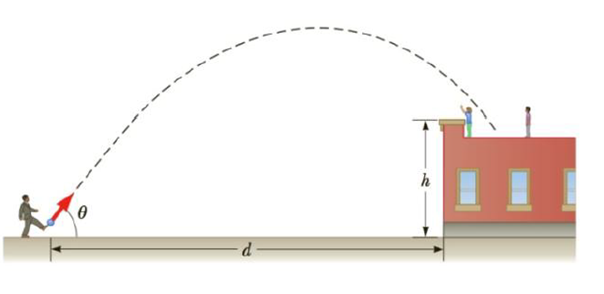Chapter 3, Problem 19P

Chapter
Section
Textbook Problem

A playground is on the flat roof of a city school, 6.00 m above the street below (Fig. P3.19). The vertical wall of the building is h = 7.00 m high, to form a 1-m-high railing around the play-ground. A ball has fallen to the street below, and a passerby returns it by launching it at an angle of θ = 53.0° above the horizontal at a point d = 24.0 m from the base of the building wall. The ball takes 2.20 s to reach a point vertically above the wall, (a) Find the speed at which the ball was launched. (b) Find the vertical distance by which the ball clears the wall. (c) Find the horizontal distance from the wall to the point on the roof where the ball lands.(a)

To determine
The launch speed of the ball.

Explanation

The horizontal displacement is,

Δx=(v0cosθ)t

If the ball travels horizontally a distance Δx in time t , the initial launch speed is,

v0=Δxtcosθ

Substitute 24.0m for Δx , 2

(b)

To determine
Thevertical distance by which the ball clears the wall.

(c)

To determine
The horizontal distance from the wall to the point on the roof where the ball lands.

Still sussing out bartleby?

Check out a sample textbook solution.

See a sample solution

The Solution to Your Study Problems

Bartleby provides explanations to thousands of textbook problems written by our experts, many with advanced degrees!

Get Started

Experimenting with portion sizes at home is a valuable exercise in self-education. T F

Nutrition: Concepts and Controversies - Standalone book (MindTap Course List)

Velocity is a(n) ___ quantity. (2.2)

An Introduction to Physical Science

How many autosomes are present in a body cell of a human being? In a gamete?

Human Heredity: Principles and Issues (MindTap Course List)